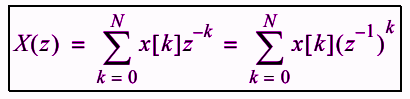# Z Transformation Formula

Time-domain and the frequency domains are considered in the study of discrete-time signals and systems. The z-transform gives us a third representation for the study. However all the three domains are related to each other. A special characteristic of the z-transform is that with respect to the signals and system of interest to us, all of the analysis will be in terms of ratios of polynomials. And we know that working with these polynomials is relatively easy.

## Definition of Z-Transform

In mathematics and signal processing, the Z-transform converts a discrete-time signal, which is a sequence of real or complex numbers, into a complex frequency-domain representation.

Also, it can be considered as a discrete-time equivalent of the Laplace transform.Where,

x[n]= Finite length signal

[0, N] = Sequence support interval

z = Any complex number

N = Integer

### Solved Problems

Example 1: Write the z-transform for a finite sequence given below.

x = {-2, -1, 1, 2, 3, 4, 5}

Solution:

Given sequence of sample numbers x[n]= is x = {-2, -1, 1, 2, 3, 4, 5}

z-transform of x[n] can be written as:

X(z) = -2z0 – z-1 + z-2 + 2z-3 + 3z-4 + 4z-5 + 5z-6

This can be further simplified as below.

X(z) = -2 – z-1 + z-2 + 2z-3 + 3z-4 + 4z-5 + 5z-6

Example 2: Write the z-transform of the following power series.

$$\begin{array}{l}f(x)=\left\{\begin{matrix} a^k,\ k\ge 0 & \\ 0,\ k< 0 & \end{matrix}\right.\end{array}$$

Solution:

Given,

$$\begin{array}{l}f(x)=\left\{\begin{matrix} a^k,\ k\ge 0 & \\ 0,\ k< 0 & \end{matrix}\right.\end{array}$$

It can be expressed using z-transform as:

$$\begin{array}{l}F(z) = \sum_{k=0}^{\infty}a^k z^{-k} \\=\sum_{k=0}^{\infty}(az^{-1})^k\\=\frac{1}{1-az^{-1}}\\=\frac{z}{z-a}\end{array}$$